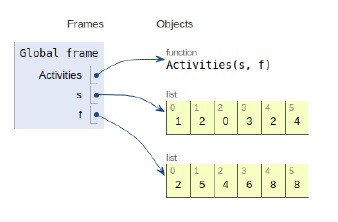# Python Program for Activity Selection Problem

Problem statementWe are given n activities with their respective starting and finish times. We need to select the maximum number of activities that can be performed by a single person, provided he works on one activity at a time.

Variable notations

N - Total number of activities

S - An array that contains start time of all activities

F - An array that contains finish time of all activities

Now let’s observe the solution in the implementation below −

# Greedy approach

## Example

Live Demo

# maximum number of activities that can be performed by a single person
def Activities(s, f ):
n = len(f)
print ("The selected activities are:")
# The first activity is always selected
i = 0
print (i,end=" ")
# For rest of the activities
for j in range(n):
# if start time is greator than or equal to that of previous activity
if s[j] >= f[i]:
print (j,end=" ")
i = j
# main
s = [1, 2, 0, 3, 2, 4]
f = [2, 5, 4, 6, 8, 8]
Activities(s, f)

## Output

The selected activities are:
0 1All the variables are declared in the local scope and their references are seen in the figure above.

## Conclusion

In this article, we have learned about how we can make a Python Program for Activity Selection Problem